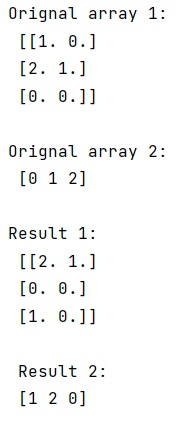# Better way to shuffle two numpy arrays in unison

In this tutorial, we will learn how to shuffle two NumPy arrays in unison? By Pranit Sharma Last updated : May 24, 2023

NumPy is an abbreviated form of Numerical Python. It is used for different types of scientific operations in python. Numpy is a vast library in python which is used for almost every kind of scientific or mathematical operation. It is itself an array which is a collection of various methods and functions for processing the arrays.

## Problem Statement

Suppose we are given two NumPy arrays of different shapes but with the same length, we need to shuffle each of them such that corresponding elements continue to correspond which means that shuffle them in unison with respect to their leading indices.

## Shuffling Two NumPy Arrays in Unison

To shuffle two NumPy arrays in unison, you can simply use the NumPy array indexing technique, where you have to first define a function inside which we will write our code for the shuffling of arrays and we will return the shuffled arrays. This method is much faster as it does create copies and uses advanced indexing. the simplicity and readability of this, and advanced indexing is a much more amazing technique for shuffling the arrays.

Let us understand with the help of an example,

## Python program to shuffle two numpy arrays in unison

```# Import numpy
import numpy as np

# Creating two numpy arrays
arr1 = np.array([[1., 0.], [2., 1.], [0., 0.]])
arr2 = np.array([0, 1, 2])

# Display original arrays
print("Orignal array 1:\n",arr1,"\n")
print("Orignal array 2:\n",arr2,"\n")

# Defining a function for shuffling
def fun(arr1, arr2):
assert len(arr1) == len(arr2)
p = np.random.permutation(len(arr1))
return arr1[p], arr2[p]

# Calling this function
res1,res2 = fun(arr1,arr2)

# Display result
print("Result 1:\n",res1,"\n\n","Result 2:\n",res2)
```

### Output